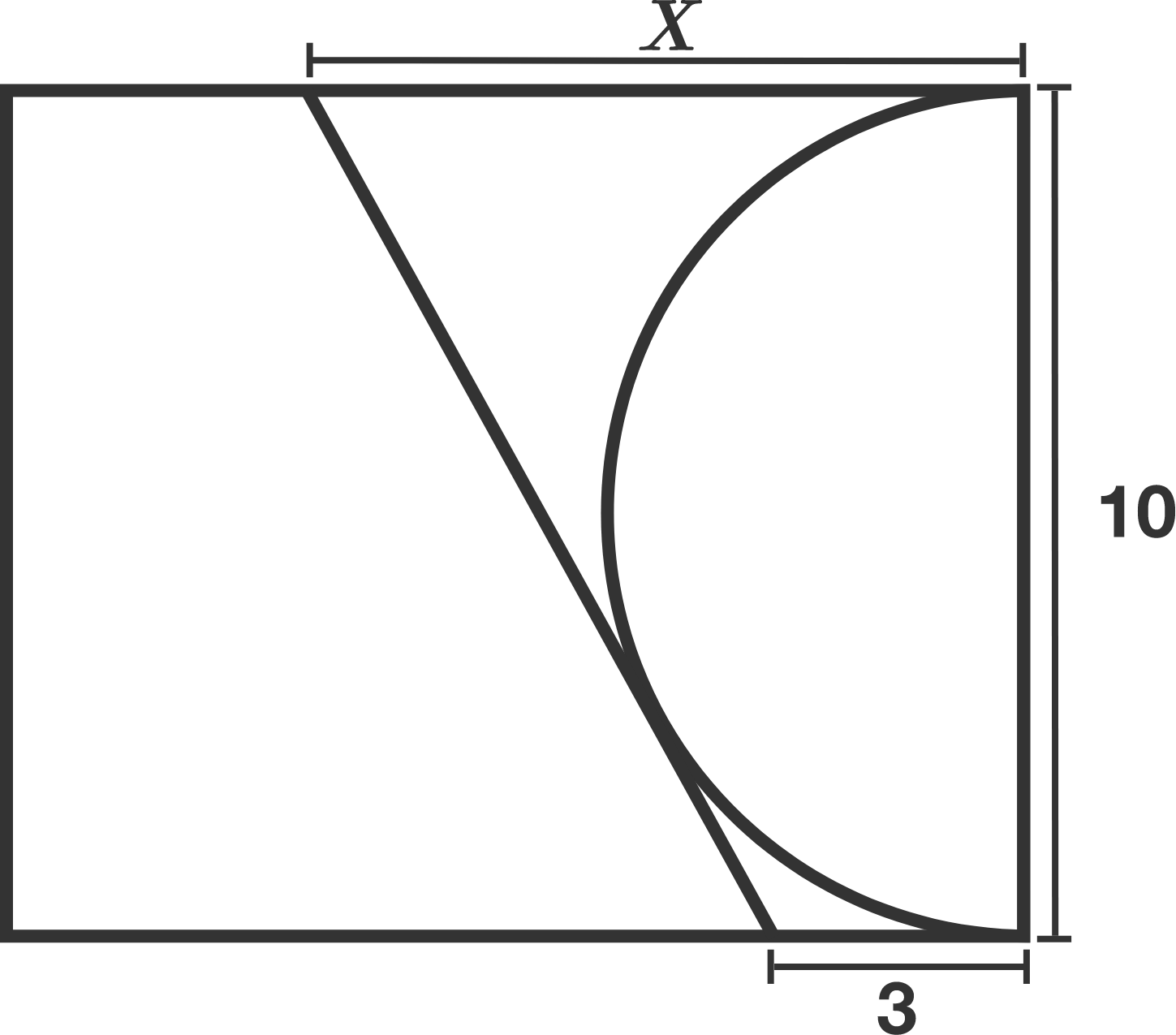# Go The Distance

Geometry Level 3A semicircle is drawn on the side with length 10 of a rectangle, as shown in the diagram above.

Then a line is drawn from the point 3 units to the left of the bottom right corner, such that it is tangent to the semicircle.

Where do this line and the upper side of the rectangle intersect?

Enter the distance $X$, correct to 2 decimal places.

×